For a full list of BASHing data blog posts see the index page.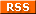# Finding one-to-many entries in a data table (updated)

The command described in this post is useful in data checking, but it's a little hard to explain what it actually does. It answers this question: Are there records with non-blank entries in field 1 that have multiple corresponding entries in field 2?

I'll start simple. The following list (filename "list") contains two comma-separated fields. There are a number of duplicates in the list, two partial blanks and several records where the same 3-letter field has a different 3-number field after it:

ddd,222
eee,111
aaa,333
ddd,555
,222
aaa,333
bbb,555
ddd,222
aaa,444
,444
eee,111
ccc,111
bbb,555
ddd,222

It'll be a little easier to see all that if I sort and uniqify "list":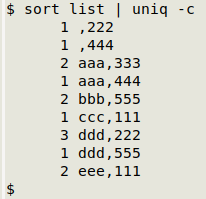What I'd like to do is select and tally the entries in "list" in which a non-blank first field has more than one partner in the second field. In other words, I want just the "aaa" and "ddd" tallies, not the blank, "bbb", "ccc" or "eee" tallies.

Here's the command I'll use, explained later in this post:

awk -F"," '\$1 != "" {a[\$1]++; b[\$1][\$2]++} \
END {for (i in b) {for (j in b[i]) \
{if (a[i]>b[i][j]) print b[i][j] "\t" i FS j}}}' list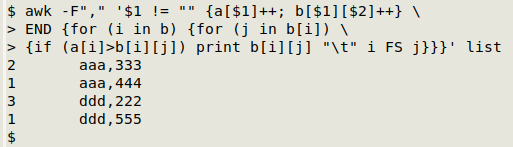Now why (you may be thinking) would I want just those "one to many" instances in a data table? Because it's a good way to find out if two fields that should always have the same pair of entries (like a customer ID number and that customer's home address in a sales contacts table) in fact have multiple second-field entries for the same first field.

I've saved this complicated command as a function, "one2many", and tweaked it for the field separator I always use, a tab:

one2many() { awk -F"\t" -v one="\$2" -v many="\$3" '\$one != "" {a[\$one]++; b[\$one][\$many]++} END {for (i in b) {for (j in b[i]) {if (a[i]>b[i][j]) print b[i][j] FS i FS j}}}' "\$1"; }

The function takes three arguments: filename, number of first field, number of second field (the field where multiple values might be found).

In my data auditing, I often check tables that have a verbal location field, a latitude (or northing) field and a longitude (or easting) field. It sometimes happens that the same location is written in several different ways in the verbal field, like "3 km W of Route 6 and Route 3 junction" and "3km west jcn Routes 6 and 3", but the same way in the coordinate fields. To find these verbal location variants I use a variant of "one2many" called "one2manyLL". I concatenate the two coordinate fields with a comma between, and use the concatenation as my first field. "one2manyLL" takes four arguments: filename, number of latitude (or northing) field, number of longitude (or easting) field and number of verbal location field:

one2manyLL() { awk -F"\t" -v lat="\$2" -v lon="\$3" -v verbal="\$4" '\$lat != "" {a[\$lat","\$lon]++; b[\$lat","\$lon][\$verbal]++} END {for (i in b) {for (j in b[i]) {if (a[i]>b[i][j]) print b[i][j] FS i FS j}}}' "\$1"; }

Here's the first set of results from "one2manyLL" in a real-world table ("jch1") which uses easting/northing for coordinates: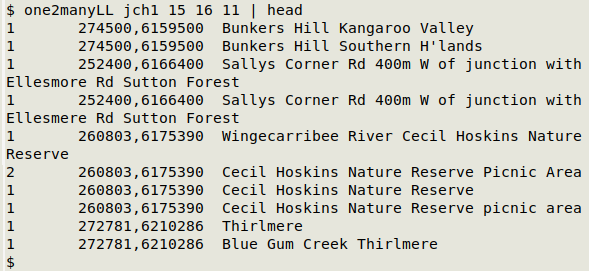Same easting (field 15) and northing (field 16) in the four cases shown, but different verbal locations (field 11).

I can also reverse the logic and look for the same verbal location with different coordinates. That's less useful to me, because a single verbal location, like "Morton National Park", might validly have multiple coordinate pairs in the table. Here's the reverse-logic command at work: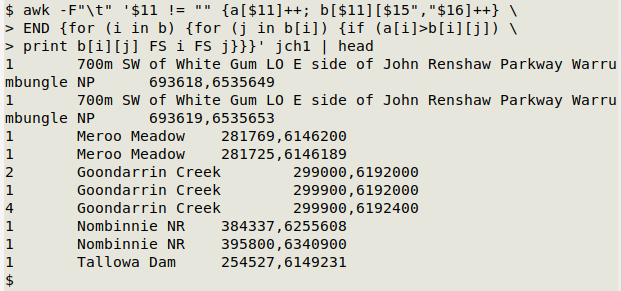Of course, the coordinates vs verbal location test (with "one2manyLL()") assumes that the coordinates are all clean and consistent! That was true in the "jch1" case, but it's something I'd check before running the test, and it would be worth checking first field entries in any data table before testing this way. For consistency checks in an individual field I use the tally function described in A Data Cleaner's Cookbook.

How it works. Let's go back to "list" and the command, which requires GNU AWK (gawk) version 4 or later:

awk -F"," '\$1 != "" {a[\$1]++; b[\$1][\$2]++} \
END {for (i in b) {for (j in b[i]) \
{if (a[i]>b[i][j]) print b[i][j] "\t" i FS j}}}' list

-F"," Tells AWK that the field separator (FS) in "list" is a comma.

\$1 != "" Restricts AWK to lines where the first field is not blank.

{a[\$1]++; b[\$1][\$2]++} This action is carried out for every line in "list". a[\$1]++ creates the array "a" and keeps a count of the different items in field 1, which in "list" is the field with 3-letter strings. b[\$1][\$2]++ creates an "array within an array" called "b". For each different item in field 1, "b" associates a sub-array with the different items in field 2 (3-number strings) that are paired with that particular field 1 item. The values for this array are counts of the different combinations (e.g. 2 of "aaa" and "333", 1 of "aaa" and "444" etc).

In the END statement, AWK walks through array "b". It does this with an "outer" for loop (for (i in b)) and an "inner" for loop (for (j in b[i])). To see what that walk finds, I'll print out the tally counts from "a", the tally counts from "b" and the field 1 ("i") and field 2 ("j") items, then sort the results by the 3-letter string items: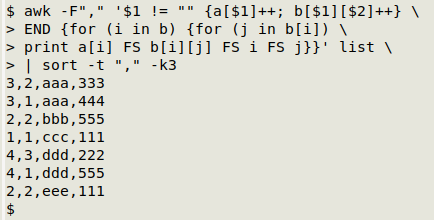Ignoring the first comma-separated field, the result is the same as you'd get with sort and uniq -c (see above). But notice that first field, which counts the total number of occurrences of the first-field item. Where there's only one pairing of fields (as in the two instances of "eee,111"), the first and second numbers are equal. Where there are multiple pairings of 3-number strings with a 3-letter string, the first comma-separated field (the count from "a[i]") is larger than the second comma-separated field (the count from "b[i][j]").

That's the test used by the command: if (a[i]>b[i][j]). If true, AWK prints the count, the first field item and the second field item with tab separation: print b[i][j] FS i FS j. If false, nothing gets printed.

Update. The two functions described here have been modified in A Data Cleaner's Cookbook so that sets of similar items are separated by a blank line. The modifications are shown and explained here.

Last update: 2022-02-20
The blog posts on this website are licensed under a